# 基于分层差分方程的热防护服热量分布规律的模拟Simulation of Heat Distribution Law of Thermal Protective Clothing Based on Lay-ered Difference Equation

DOI: 10.12677/MOS.2019.81002, PDF, HTML, XML, 下载: 620  浏览: 1,017

Abstract: In order to study the heat distribution of heat-resistant garments in high-temperature environ-ments and further to reduce the production cost of heat-resistant garments under high-temperature environment, and shorten the development cycle, in this paper, we first establish a model of high-temperature protective clothing-air-skin for different material layers. The heat conduction equation is used to study the heat distribution of different layers, where the thermal diffusivity in the heat transfer equation depends on the parameter values of the dielectric material. We propose two models to solve the boundary condition problem in partial differential equations. The concept of thermal resistance is introduced in Model I. According to the Crank-Nicolson implicit scheme for solving partial differential equations in numerical analysis, the temperature-space-time correlation results in different material layers can be obtained. In Model II, four different material layers are considered as a whole. Thus, the classical heat differential equations are used to solve the partial differentials of the heat conduction equations for the four layers. Numerical solution of the equation shows that the methods are workable.

1. 引言

2. 分层热传导模型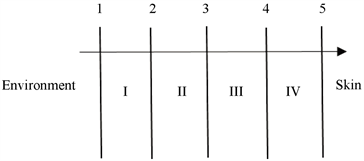Figure 1. Schematic diagram of thermal conduction model(1)

3. 模型的求解方法

3.1. 基于热阻法求解边界条件以及Crank-Nicolson隐式格式求解偏微分方程

${T}_{i}\left(x,t\right)=\left({T}_{i}\left(1,1\right),\cdots ,{T}_{i}\left(Ax-2,1\right),{T}_{i}\left(1,2\right),\cdots {T}_{i}\left(Ax-2,2\right),\cdots {T}_{i}\left(1,Bt-1\right),\cdots ,{T}_{i}\left(Ax-2,Bt-1\right)\right)$ (2)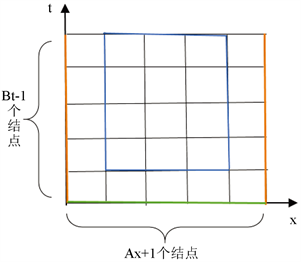Figure 2. Implicit schematic diagram of Crank-Nicolson

$\begin{array}{l}-\frac{1}{2}r{T}_{i}\left(x-1,t+1\right)+\left(1+r\right){T}_{i}\left(x,t+1\right)-\frac{1}{2}r{T}_{i}\left(x+1,t+1\right)\\ =\frac{1}{2}r{T}_{i}\left(x-1,t\right)+\left(1-r\right){T}_{i}\left(x,t\right)+\frac{1}{2}r{T}_{i}\left(x+1,t\right)\end{array}$ (3)

$\begin{array}{l}\left(\begin{array}{cccc}1+2r& -r& \cdots & 0\\ -r& 1+2r& \ddots & ⋮\\ 0& \ddots & \ddots & -r\\ 0& 0& -r& 1+2r\end{array}\right)\cdot \left(\begin{array}{c}{T}_{i}\left(1,t+1\right)\\ {T}_{i}\left(2,t+1\right)\\ ⋮\\ {T}_{i}\left(Ax-2,t+1\right)\end{array}\right)\\ =\left(\begin{array}{cccc}1+2r& -r& \cdots & 0\\ -r& 1+2r& \ddots & ⋮\\ 0& \ddots & \ddots & -r\\ 0& 0& -r& 1+2r\end{array}\right)\cdot \left(\begin{array}{c}{T}_{i}\left(1,t\right)\\ {T}_{i}\left(2,t\right)\\ ⋮\\ {T}_{i}\left(Ax-2,t\right)\end{array}\right)\\ +\left(\begin{array}{c}0.5r{T}_{i}\left(0,t\right)+0.5r{T}_{i}\left(0,t+1\right)\\ 0\\ ⋮\\ 0.5r{T}_{i}\left(Ax-2,t\right)+0.5r{T}_{i}\left(Ax-2,t+1\right)\end{array}\right)\end{array}$ (4)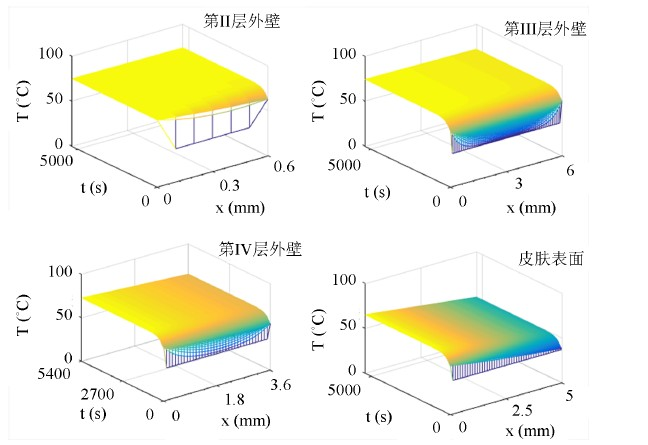Figure 3. Temperature-time-space Distribution of Layer I-IV Based on the Implicit Method of Solving Partial Differential Equation of CN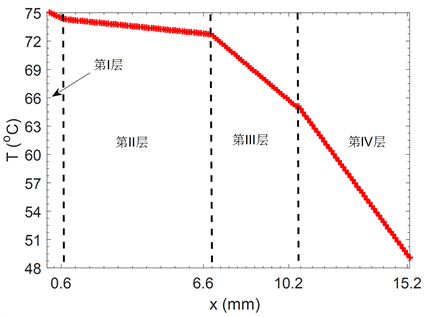Figure 4. The change of temperature at the last moment (5400 s) with the position based on the method of solving partial differential equation with CN implicit scheme

3.2. 基于整体化法以及古典显式差分法求解偏微分方程

$\frac{{T}_{i}\left(x,t+1\right)-{T}_{i}\left(x,t\right)}{k}-\frac{{T}_{i}\left(x+1,t\right)-2{T}_{i}\left(x,t\right)+{T}_{i}\left(x-1,t\right)}{{h}^{2}}=0$

${T}_{i}\left(x,t+1\right)=r{T}_{i}\left(x-1,t\right)+\left(1-2r\right){T}_{i}\left(x,t\right)+r{T}_{i}\left(x+1,t\right)$ (5)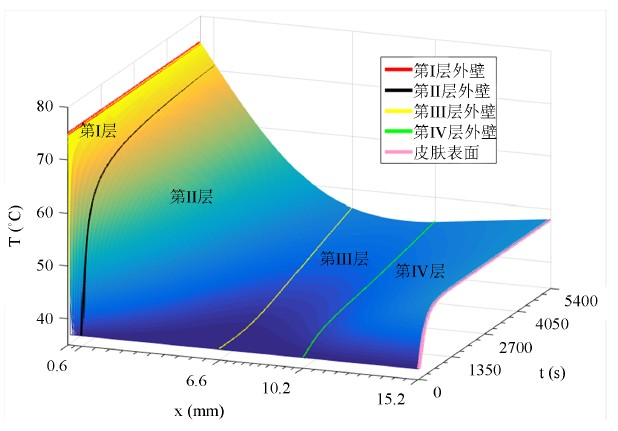Figure 5. Temperature-time-space distribution of layer I-IV based on classical explicit partial differential equation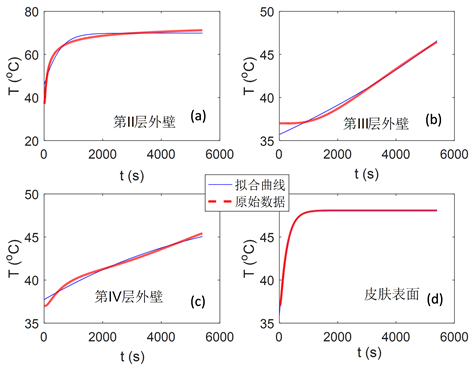Figure 6. On the basis of the classical explicit partial differential equation solution method, the temperature change of the boundary layer I-IV with time

4. 模型检验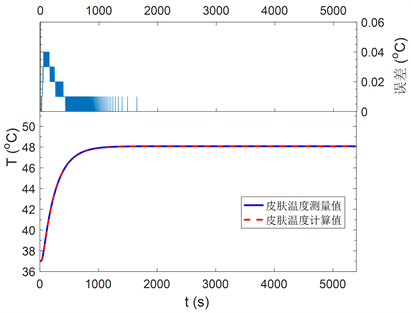Figure 7. The calculation and measurement of skin temperature and its error based on the method of solving implicit partial differential equation of CN

5. 结语

NOTES

*通讯作者。

  Liu, J. (2002) Numerical Solution of Forward and Backward Problem for 2-D Heat Conduction Equation. Journal of Computational & Applied Mathematics, 145, 459-482. https://doi.org/10.1016/S0377-0427(01)00595-7  张海峰, 葛新石, 叶宏. 预测复合材料导热系数的热阻网络法[J]. 功能材料, 2005, 36(5): 757-759.  熊平, 艾红雷, 卢涛, 等. 一维非稳态导热反问题反演管道内壁面温度波动[J]. 核动力工程, 2018, 39(2): 96-100.  赵兰萍, 徐烈. 固体界面间接触导热的机理和应用研究[J]. 低温工程, 2000(4): 29-29.  孙鸿烈. 解一维热传导方程的高精度的隐式差分格式[J]. 数学的实践与认识, 1998(3): 197-200.  http://www.mcm.edu.cn/html_cn/node/7cec7725b9a0ea07b4dfd175e8042c33.html  金承日. 解抛物型方程的高精度显式差分格式[J]. 计算数学, 1991, 13(1): 38-44.  张小峰, 张红武. Crank-Nicolson格式精度的改进[J]. 水科学进展, 2001, 12(1): 33-38.  马继涌, 谢鸣. 多层壁导热反问题的解析公式及其应用[J]. 哈尔滨建筑大学学报, 1995(6): 71-75.  潘斌. 热防护服装热传递数学建模及参数决定反问题[D]: [硕士学位论文]. 杭州: 浙江理工大学, 2017.